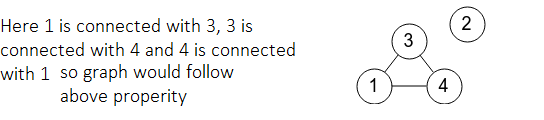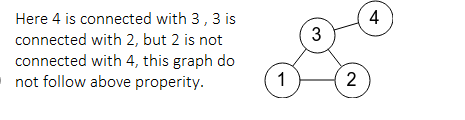# Check for transitive property in a given Undirected Graph

• Difficulty Level : Easy
• Last Updated : 15 Sep, 2021

Given an undirected graph G with vertices numbered in the range [1, N] and an array Edges[][] consisting of M edges, the task is to check if all triplets of the undirected graph satisfies the transitive property or not. If found to be true, then print “YES”. Otherwise, print “NO”.

Transitive property of an undirected graph states that:
If vertex X is connected to vertex Y, vertex Y is connected to vertex Z, then vertex X must be connected to the vertex Z.

Examples:

Input: N = 4, M = 3 Edges[] = {{1, 3}, {3, 4}, {1, 4}}
Output: YES
Explanation:Input : N = 4, M = 4, Edges[] = {{3, 1}, {2, 3}, {3, 4}, {1, 2}}
Output: NO
Explanation:Naive Approach: The simplest approach to solve the above problem is to traverse over every triplet of vertices (i, j, k), and for each such triplet, check if there is an edge between vertices j and k if i and j, and i and k are directly connected by an edge with the help of an adjacency matrix.

Time Complexity: O(N3)
Auxiliary Space: O(N2)

Efficient Approach: The idea is to find all the connected components present in the graph. Finally, check if all the connected components of the graph are a complete graph or not. If found to be true, then print “YES”. Otherwise, print “NO”. Follow the steps below to solve the problem:

• Represent the graph, G in the form of adjacency list.
• Find all the connected components of the graph and check if the connected component is a complete graph or not by performing the following operations:
• Find total count of vertices in the current connected graph say, X.
• If all the vertices of the connected component are not connected to X – 1 vertices, then print “NO”.
• Finally, check if all the connected components are a complete graph or not. IF found to be true, then print “YES”.

Below is the implementation of the above approach:

## C++

 `// C++ program of the above approach``#include ``using` `namespace` `std;` `// Stores undirected graph using``// adjacency list representation``class` `Graph {` `    ``// Stores count of vertices``    ``int` `V;` `    ``// adj[i]: Store all the nodes``    ``// connected to i``    ``list<``int``>* adj;` `    ``// DFS function``    ``void` `DFSUtil(``int` `v, ``bool` `visited[], ``int` `id[],``                 ``int` `id_number, ``int``& c);` `public``:``    ``Graph(``int` `V);``    ``~Graph();` `    ``// Connect two vertices v and w``    ``void` `addEdge(``int` `v, ``int` `w);` `    ``// Check if the connected component``    ``// is a complete graph or not``    ``bool` `connectedComponents();``};` `Graph::Graph(``int` `V)``{``    ``this``->V = V;``    ``adj = ``new` `list<``int``>[V + 1];``}` `// Destructor``Graph::~Graph() { ``delete``[] adj; }` `// Function to add an undirected``// edge between two vertices``void` `Graph::addEdge(``int` `v, ``int` `w)``{``    ``adj[v].push_back(w);``    ``adj[w].push_back(v);``}` `// Function to find all the connected``// components of a graph using DFS``void` `Graph::DFSUtil(``int` `v, ``bool` `visited[], ``int` `id[],``                    ``int` `id_number, ``int``& c)``{` `    ``// Mark the vertex v as visited``    ``visited[v] = ``true``;` `    ``// Assign an id of current``    ``// connected component``    ``id[v] = id_number;` `    ``// Increase the count of vertices in``    ``// current connected component``    ``c++;` `    ``// Recursively call for all the``    ``// vertices adjacent to this vertex``    ``list<``int``>::iterator i;` `    ``// Iterate over all the adjacent``    ``// vertices of the current vertex``    ``for` `(i = adj[v].begin(); i != adj[v].end();``         ``++i) {` `        ``// If current vertex is not visited``        ``if` `(!visited[*i])``            ``DFSUtil(*i, visited, id,``                    ``id_number, c);``    ``}``}` `// Function to find connected``// components of the graph``bool` `Graph::connectedComponents()``{` `    ``bool``* visited = ``new` `bool``[V + 1];` `    ``// id[i]: Stores an unique id of connected``    ``// component in which vertex i exists``    ``int``* id = ``new` `int``[V + 1];` `    ``// Store count of nodes in current``    ``// connected component``    ``int``* component_size = ``new` `int``[V + 1];` `    ``// Mark all the vertices as not visited``    ``for` `(``int` `v = 1; v <= V; v++)``        ``visited[v] = ``false``;` `    ``for` `(``int` `v = 1; v <= V; v++) {` `        ``// If vertex v is not marked``        ``if` `(visited[v] == ``false``) {` `            ``// Stores the size of a component``            ``// in which vertex v lies``            ``int` `c = 0;` `            ``// Stores id of current``            ``// connected component``            ``int` `id_number = v;` `            ``DFSUtil(v, visited, id,``                    ``id_number, c);` `            ``// Stores count of vertices of``            ``// current component``            ``component_size[v] = c;``        ``}``        ``else` `{` `            ``component_size[v]``                ``= component_size[id[v]];``        ``}``    ``}` `    ``// Iterate over all the vertices``    ``for` `(``int` `v = 1; v <= V; v++) {` `        ``// IF connected component[v] is``        ``// not a complete graph``        ``if` `(component_size[v] - 1``            ``!= (``int``)adj[v].size()) {` `            ``delete``[] visited;``            ``return` `false``;``        ``}``    ``}` `    ``delete``[] visited;``    ``return` `true``;``}` `// Function to check if graph is``// Edge Transitive or not``void` `isTransitive(``int` `N, ``int` `M,``                  ``vector > Edge)``{` `    ``// Initialize a graph``    ``Graph G(N);` `    ``// Traverse the array Edge[]``    ``for` `(``int` `i = 0; i < M; i++) {``        ``G.addEdge(Edge[i], Edge[i]);``    ``}` `    ``// If all the connected components``    ``// are a complete graph``    ``int` `f = G.connectedComponents();``    ``if` `(f == 0) {``        ``cout << ``"NO\n"``;``    ``}``    ``else` `{``        ``cout << ``"YES\n"``;``    ``}``}` `// Driver Code``int` `main()``{``    ``// Input``    ``int` `N = 4, M = 3;``    ``vector > Edge{ { 1, 3 },``                               ``{ 3, 4 },``                               ``{ 1, 4 } };``    ``isTransitive(N, M, Edge);` `    ``return` `0;``}`

## Python3

 `# Python3 program of the above approach` `# Function to add an undirected``# edge between two vertices``def` `addEdge(v, w):``    ``global` `adj``    ``adj[v].append(w)``    ``adj[w].append(v)` `# Function to find all the connected``# components of a graph using DFS``def` `DFSUtil(v, ``id``, id_number):``    ``global` `visited, adj, c` `    ``# Mark the vertex v as visited``    ``visited[v] ``=` `True` `    ``# Assign an id of current``    ``# connected component``    ``id``[v] ``=` `id_number` `    ``# Increase the count of vertices in``    ``# current connected component``    ``c ``+``=` `1` `    ``# Iterate over all the adjacent``    ``# vertices of the current vertex``    ``for` `i ``in` `adj[v]:``      ` `        ``# If current vertex is not visited``        ``if` `(``not` `visited[i]):``            ``DFSUtil(i, ``id``, id_number)` `# Function to find connected``# components of the graph``def` `connectedComponents():``    ``global` `V, adj, visited, c` `    ``# id[i]: Stores an unique id of connected``    ``# component in which vertex i exists``    ``id` `=` `[``0``]``*``(V ``+` `1``)` `    ``# Store count of nodes in current``    ``# connected component``    ``component_size ``=` `[``0``]``*``(V ``+` `1``)``    ``for` `v ``in` `range``(``1``, V ``+` `1``):` `        ``# If vertex v is not marked``        ``if` `(visited[v] ``=``=` `False``):` `            ``# Stores the size of a component``            ``# in which vertex v lies``            ``c ``=` `0` `            ``# Stores id of current``            ``# connected component``            ``id_number ``=` `v``            ``DFSUtil(v, ``id``, id_number)` `            ``# Stores count of vertices of``            ``# current component``            ``component_size[v] ``=` `c``        ``else``:` `            ``component_size[v] ``=` `component_size[``id``[v]]` `    ``# Iterate over all the vertices``    ``for` `v ``in` `range``(``1``, V ``+` `1``):` `        ``# IF connected component[v] is``        ``# not a complete graph``        ``if` `(component_size[v] ``-` `1` `!``=` `len``(adj[v])):``            ``return` `False``    ``return` `True` `# Function to check if graph is``# Edge Transitive or not``def` `isTransitive(N, M, Edge):``    ``global` `adj, visited, c` `    ``# Traverse the array Edge[]``    ``for` `i ``in` `range``(M):``        ``addEdge(Edge[i][``0``], Edge[i][``1``])` `    ``# If all the connected components``    ``# are a complete graph``    ``f ``=` `connectedComponents()``    ``if` `(f ``=``=` `0``):``        ``print``(``"NO"``)``    ``else``:``        ``print``(``"YES"``)` `# Driver Code``if` `__name__ ``=``=` `'__main__'``:``    ` `    ``# Input``    ``V, c ``=` `5``, ``0``    ``adj ``=` `[[] ``for` `i ``in` `range``(V ``+` `1``)]``    ``visited ``=` `[``False``] ``*` `(V ``+` `1``)` `    ``N, M ``=` `4``, ``3``    ``Edge ``=` `[ [ ``1``, ``3` `],``           ``[ ``3``, ``4` `],``           ``[ ``1``, ``4` `] ]``    ``isTransitive(N, M, Edge)` `    ``# This code is contributed by mohit kumar 29`

Output:

`YES`

Time Complexity: O(N + M)
Auxiliary Space: O(N2)

My Personal Notes arrow_drop_up